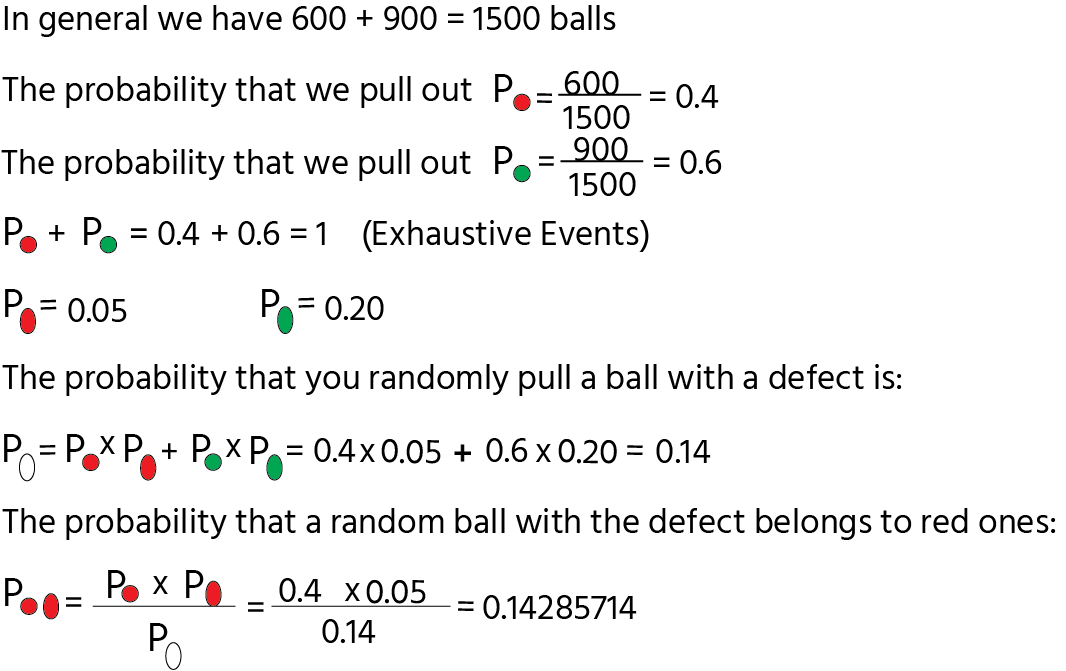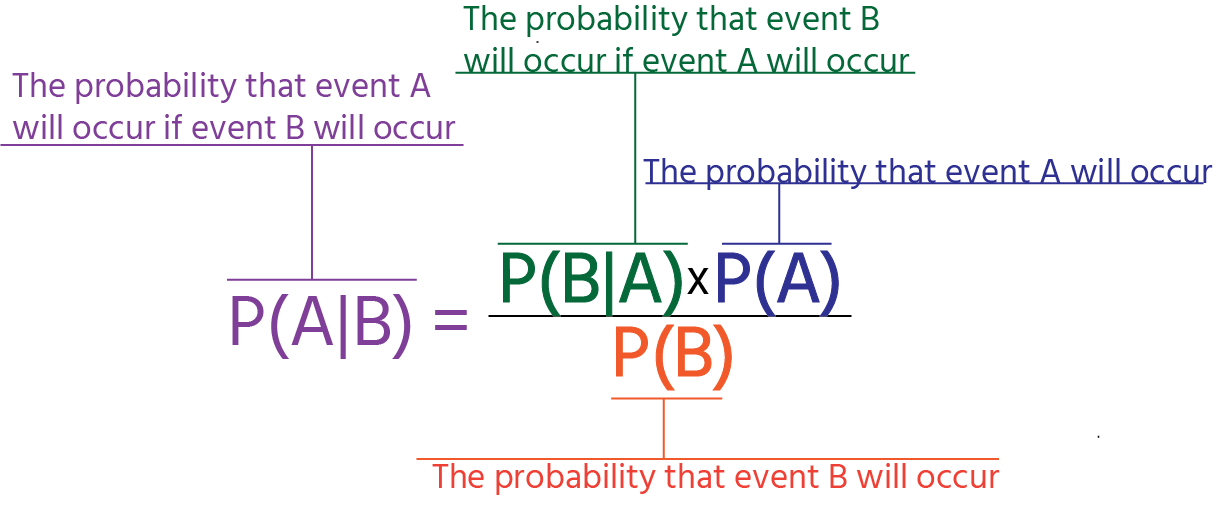Course Content

Probability TheoryBayes Theorem

Let's talk about the Bayes theorem!

What is it?

The theorem helps figure out the probability that one event will happen, considering that another statistically related one happened.

I strongly believe that everything is clear only with examples, so let's move to an example.

Example:

Let's assume that we have a box with red and green balls. There are 600 red balls and 900 green balls. We know that among all balls, 5% of red balls have a defect and 20% of green balls have a defect too. Randomly we pulled out a ball with a defect, calculate the probability that it relates to the red ones.It should be noted that this theorem is widely used in the data science; therefore, look to the general formula:Assume that you have a box with red and green balls. There are `600` red balls and `900` green balls. You know that among all balls `5`% of red balls have a defect and `20`% of green balls have a defect too. Randomly you pulled out a ball with a defect; calculate the probability that it relates to the green ones.